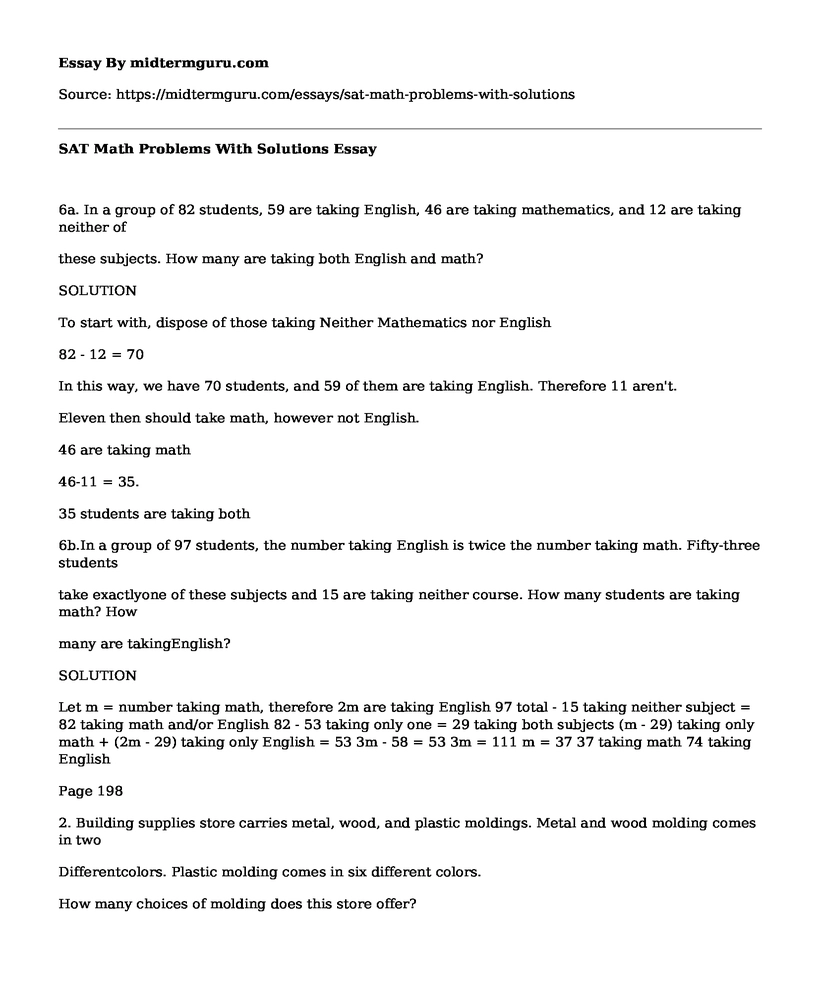# SAT Math Problems With Solutions

 Paper Type: Pages:  3 Wordcount:  689 Words Date:  2021-05-25
Categories:

6a. In a group of 82 students, 59 are taking English, 46 are taking mathematics, and 12 are taking neither of

Is your time best spent reading someone else’s essay? Get a 100% original essay FROM A CERTIFIED WRITER!

these subjects. How many are taking both English and math?

SOLUTION

82 - 12 = 70

In this way, we have 70 students, and 59 of them are taking English. Therefore 11 aren't.

Eleven then should take math, however not English.

46 are taking math

46-11 = 35.

35 students are taking both

6b.In a group of 97 students, the number taking English is twice the number taking math. Fifty-three students

take exactlyone of these subjects and 15 are taking neither course. How many students are taking math? How

many are takingEnglish?

SOLUTION

Let m = number taking math, therefore 2m are taking English 97 total - 15 taking neither subject = 82 taking math and/or English 82 - 53 taking only one = 29 taking both subjects (m - 29) taking only math + (2m - 29) taking only English = 53 3m - 58 = 53 3m = 111 m = 37 37 taking math 74 taking English

Page 198

2. Building supplies store carries metal, wood, and plastic moldings. Metal and wood molding comes in two

Differentcolors. Plastic molding comes in six different colors.

How many choices of molding does this store offer?

SOLUTION

Lets assume that they are counting every color.

Metal =2

Wood=2

Plastic=6

Hence making this to be 2+2+6=10

b. If each kind and each color of molding come in four different lengths, how many choices does the consumer have in

the purchase of one piece of molding?

SOLUTION

Metal = 4(lengths) x 1 (color) = 1(color) X 4(lengths) = 8 choices

Wood=4(lengths) x 2 (color) = 8choices

Plastic=4(lengths) x 6 (color) =24 choices

8+8+24 =40 total choices

8. From a standard deck of 52 playing cards, in how many ways can you draw

a. a heart or a spade?

SOLUTION

17

b. an ace or a king?

SOLUTION

213

a card numbered 2 through 10?

SOLUTION

14

a card numbered 2 through 10 or a king?

SOLUTION

8

14. There are three different roads from Cupids to Harbour Grace and five different roads from Harbour Grace to Hearts

Desire.

How many different routes are there from Cupids to Hearts Desire via Harbour Grace?

SOLUTION

72

b. How many different round trips are there from Cupids to Hearts Desire and back, passing through HarbourGrace

each way?

SOLUTION

219

Repeat (b) if you dont want to drive on any road more than once.

SOLUTION

Here, there are 15 methods that one can get the Heart Desires. You get to repeat the route used in getting the Habour Grace. Therefore, we have four choices. Then, we can't repeat the route that was used between Cupid and Habour Grace thus; we have two options. The total is 15x4x2 =120

Page 202

4.In a group of 100 people, several will have their birthdays in the same month. At least how many must have birthdays

in the same month? Why?

SOLUTION

: 10012 = 9

8. Thirty buses are to be used to transport 2000 refugees from Gander to St. Johns, Newfoundland. Each bus has 80 seats.

Assume one seat per passenger.

Prove that one of the buses will carry at least 67 passengers.

SOLUTION

Assume every one of the 30 buses conveyed 66 or fewer passengers.

At that point, the aggregate number of passengerscarried must be

less than or equivalent to 30 x 66 = 1980. Thus =, so as to

transport each of the 2000 travelers, no less than one transport must

convey no less than 67 travelers.

Prove that one of the buses will have at least 14 empty seats.

SOLUTION

Assume that the greater part of the buses had at most 13 empty seats.

At that point, every bus conveyed no less than 80 - 13 = 67 travelers.

Therefore, each of the 30 means of transport transported no less than 30 X67 = 2010 passengers.

Since that is impractical, no less than one bus had at least 14 or more empty seats.

9. In a gathering of 30 people, there are 104 different pairs of individuals who know each other.

a. Show that some person must have at least seven acquaintances.

SOLUTION

Crate a box for every individual and put into that box a checkmark for every associate with that person. 208 checkmarks need to be entered into these boxes, so some needs to contain at least = 7 checkmarks.

Show that some person must have fewer than seven acquaintances.

SOLUTION

This would mean no less than 30(7) = 210 requested sets of individuals who know each other; that is, in the 210, Edgar and Herb are counted twice, once because Herb knows Edgar and once because Edgar knows Herd. Thus, we get no less than 12 (210) = 105 sets of acquaintances.Free essays can be submitted by anyone,

so we do not vouch for their quality

Want a quality guarantee?
Order from one of our vetted writers instead

If you are the original author of this essay and no longer wish to have it published on the midtermguru.com website, please click below to request its removal:

Liked this essay sample but need an original one?

Hire a professional with VAST experience!